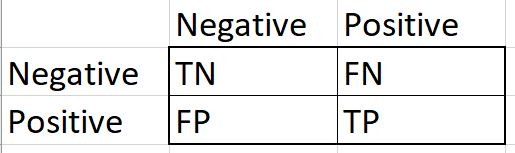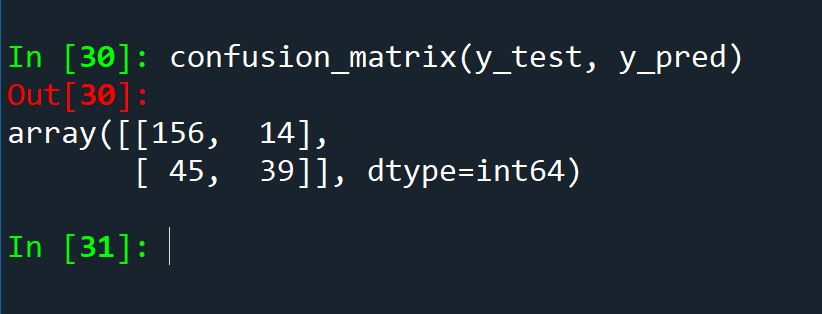# Python: Confusion Matrix

## What is a confusion matrix?

A confusion matrix is a supervised machine learning evaluation tool that provides more insight into the overall effectiveness of a machine learning classifier. Unlike a simple accuracy metric, which is calculated by dividing the number of correctly predicted records by the total number of records, confusion matrices return 4 unique metrics for you to work with.

While I am not saying accuracy is always misleading, there are times, especially when working with examples of imbalanced data,  that accuracy can be all but useless.

Let’s consider credit card fraud. It is not uncommon that given a list of credit card transactions, that a fraud event might make up a little as 1 in 10,000 records. This is referred to a severely imbalanced data.  Now imaging a simple machine learning classifier running through that data and simply labeling everything as not fraudulent. When you checked the accuracy, it would come back as 99.99% accurate. Sounds great right? Except you missed the fraud event, the only reason to try to create the model in the first place.

A confusion matrix will show you more details, letting you know that you completely missed the fraud event. Instead of a single number result, a confusion matrix provides you will 4 metrics to evaluate. (note: the minority class – (in the case of fraud – the fraudulent events) – are labeled positive by confusion matrices. So a non-fraud event is a negative. This is not a judgement between the classes, only a naming convention)

TP = true positive – minority class (fraud) is correctly predicted as positive

FP = false positive – majority class (not fraud) is incorrectly predicted

FN = false negative – minority class (fraud) incorrectly predicted

TN = true negative – majority class (not fraud) correctly predicted

In matrix form:To run a confusion matrix in Python, Sklearn provides a method called confusion_matrix(y_test, y_pred)

y_test = actual results from the test data set

y_pred = predictions made by model on test data set

so in a pseudocode example:

```model.fit(X,y)
y_pred = model.predict(X_test)```

If this is at all confusing, refer to my Python SVM lesson where I create the training and testing set and run a confusion matrix (Python: Support Vector Machine (SVM))

To run a confusion matrix in Python, first run a model, then run predictions (as shown above) and then follow the code below:

```from sklearn.metrics import confusion_matrix
confusion_matrix(y_test, y_pred)```

Output looks like this:Now, if you want to capture the TP, TN, FP, FN into individual variables to work with, you can add the ravel() function to your confusion matrix:

`TN,FP,FN,TP = confusion_matrix(y_test, y_pred).ravel()`

Thank you for taking the time to read this, and good luck on your analytics journey.